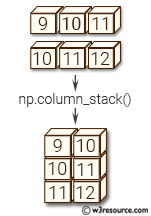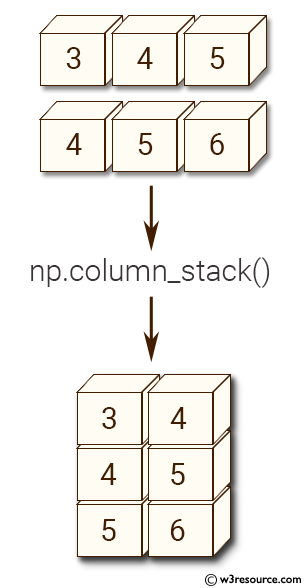# NumPy Array manipulation: column_stack() function

## numpy.column_stack() function

Stack 1-D arrays as columns into a 2-D array.
Take a sequence of 1-D arrays and stack them as columns to make a single 2-D array. 2-D arrays are stacked as-is, just like with hstack. 1-D arrays are turned into 2-D columns first.

Syntax:

`numpy.column_stack(tup)`Version: 1.15.0

Parameter:

Name Description Required /
Optional
tup Arrays to stack. All of them must have the same first dimension. Required

Return value:

stacked : 2-D array The array formed by stacking the given arrays.

Example: numpy.column_stack()

``````>>> import numpy as np
>>> x = np.array((3,4,5))
>>> y = np.array((4,5,6))
>>> np.column_stack((x,y))
array([[3, 4],
[4, 5],
[5, 6]])
``````

Pictorial Presentation:Python - NumPy Code Editor:

Previous: stack()
Next: dstack()

﻿